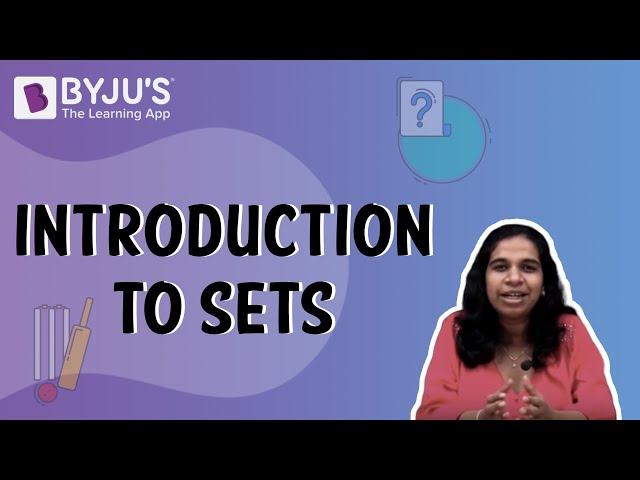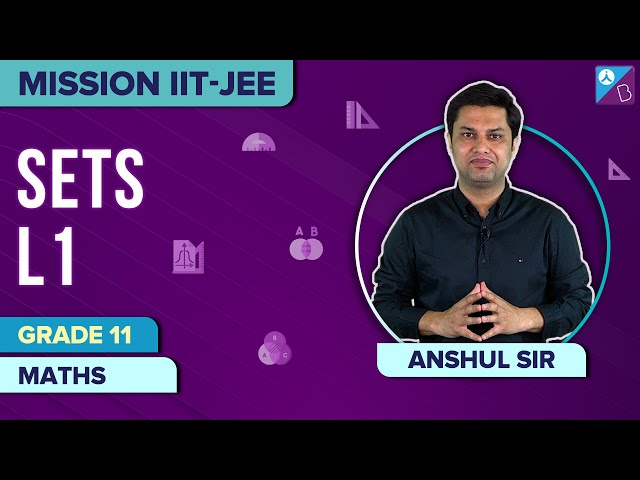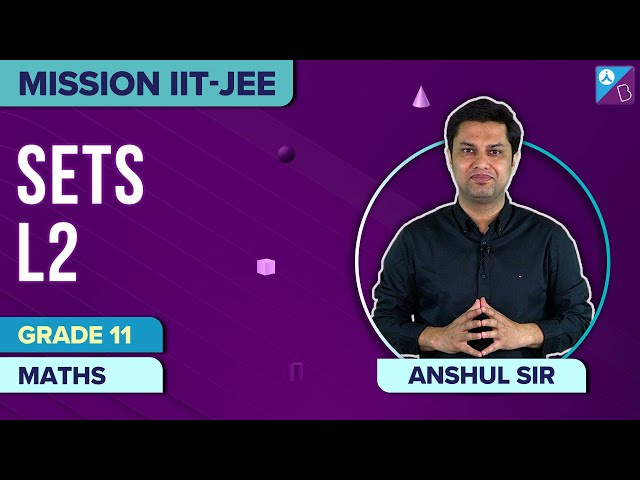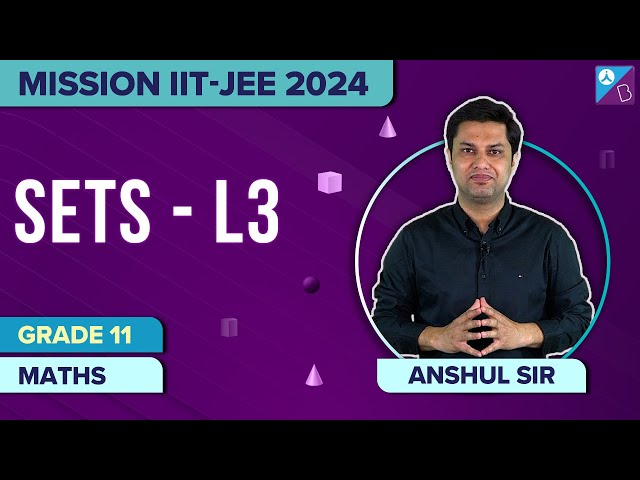# Sets For Class 11

Before learning sets for class 11, let us know first what is a set. A set is a well-defined collection of objects, whose elements are fixed and cannot vary. It means set doesn’t change from person to person. Like for example, the set of natural numbers up to 7 will remain the same as {1,2,3,4,5,6,7}. Still, if we say the set of best players in a football team, then the name of footballers could vary every time we ask about the best players, as each person has its own choice to consider the best player. Similarly, if we speak about the set of rivers in India, the elements of the set will remain the same. So, this is a real-life example of a set. In mathematics, we represent the sets in curly brackets { }.

## Introduction to SetsHere, in this chapter of sets for class 11, we will explain the types of sets along with examples.

## Types of Sets

Let us discuss the types of sets here:

### Empty Sets

The set with no elements or null elements is called an empty set. This is also called a Null set or Void set. It is denoted by {}.

For example: Let, Set X = {x:x is the number of students studying in Class 6th and Class 7th}

Since we know a student cannot learn simultaneously on two classes, therefore set X is an empty set.

### Singleton Set

The set which has only one element is called a singleton set.

For example, Set X = { 2 } is a singleton set.

### Finite and Infinite Sets

Finite sets are the one which has a finite number of elements, and infinite sets are those whose number of elements cannot be estimated, but it has some figure or number, which is very large to express in a set.

For example, Set X = {1, 2, 3, 4, 5} is a finite set, as it has a finite number of elements in it.

Set Y = {Number of Animals in India} is an infinite set, as there is an approximate number of Animals in India, but the actual value cannot be expressed, as the numbers could be very large.

### Equal Sets

Two sets X and Y are said to be equal if every element of set X is also the elements of set Y and if every element of set Y is also the elements of set X. It means set X and set Y have the same elements, and we can denote it as;

X = Y

For example, Let X = { 1, 2, 3, 4} and Y = {4, 3, 2, 1}, then X = Y

And if X = {set of even numbers} and Y = { set of natural numbers} the X ≠ Y, because natural numbers consist of all the positive integers starting from 1, 2, 3, 4, 5 to infinity, but even numbers starts with 2, 4, 6, 8, and so on.

### Subsets

A set X is said to be a subset of set Y if the elements of set X belongs to set Y, or you can say each element of set X is present in set Y. It is denoted with the symbol as X ⊂ Y.

We can also write the subset notation as;

X ⊂ Y if a ∊ X ⟹  a ∊ Y

Thus, from the above equation, “X is a subset of Y if a is an element of X implies that a is also an element of Y”.

Each set is a subset of its own set, and a null set or empty set is a subset of all sets.

### Power Sets

The power set is nothing but the set of all subsets. Let us explain how.

We know the empty set is a subset of all sets and every set is a subset of itself. Taking an example of set X = {2, 3}. From the above given statements we can write,

{} is a subset of {2, 3}

{2} is a subset of {2, 3}

{3} is a subset of {2, 3}

{2, 3} is also a subset of {2, 3}

Therefore, power set of X = {2, 3},

P(X) = {{},{2},{3},{2,3}}

### Universal Sets

A universal set is a set which contains all the elements of other sets. Generally, it is represented as ‘U’.

For example; set X = {1, 2, 3}, set Y = {3, 4, 5, 6} and Z = {5, 6, 7, 8, 9}

Then, we can write universal set as, U = {1, 2, 3, 4, 5, 6, 7, 8, 9,}

Note: From the definition of the universal set, we can say, all the sets are subsets of the universal set. Therefore,

X ⊂ U

Y ⊂ U

And Z ⊂ U

### Union of sets

A union of two sets has all their elements. It is denoted by ⋃.

For example, set X = {2, 3, 7} and set Y = { 4, 5, 8}

Then union of set X and set Y will be;

X ⋃ Y = {2, 3, 7, 4, 5, 8}

Properties of Union of Sets:

X ⋃ Y = Y ⋃ X ; Commutative law

(X ⋃ Y) ⋃ Z = X ⋃ (Y ⋃ Z)

X ⋃ {} = X ; {} is the identity of ⋃

X ⋃ X = X

U ⋃ X = U

### Intersection of Sets

Set of all elements, which are common to all the given sets, gives intersection of sets. It is denoted by the symbol ⋂.

For example, set X = {2, 3, 7} and set Y = {2, 4, 9}

So, X ⋂ Y = {2}

### Difference of Sets

The difference of set X and set Y is such that, it has only those elements which are in the set X and not in the set Y.

i.e. X – Y = {a: a ∊ X and a ∉ Y}

In the same manner, Y – X = {a: a ∊ Y and a ∉ X}

For example, if set X = {a, b, c, d} and Y = {b, c, e, f} then,

X – Y = {a, d} and Y – X = {e, f}

### Disjoint Sets

If two sets X and Y have no common elements, and their intersection results in zero(0), then set X and Y are called disjoint sets.

It can be represented as; X ∩ Y = 0

## Video Lessons on Sets

#### Sets- L1#### Sets- L2#### Sets- L3